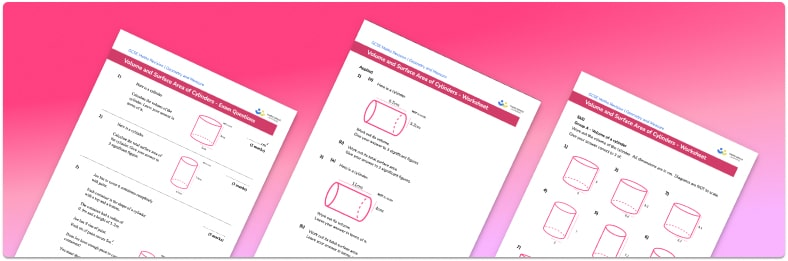# Volume And Surface Area Of Cylinders WorksheetHelp your students prepare for their Maths GCSE with this free volume and surface area of cylinder worksheet of 34 questions and answers

• Section 1 of the volume and surface area of cylinder worksheet contains 27 skills-based volume and surface area of cylinder practice questions, in 3 groups to support differentiation
• Section 2 contains 4 applied volume and surface area of cylinder questions with a mix of word problems and deeper problem solving questions
• Section 3 contains 3 foundation and higher level GCSE exam style volume and surface area of cylinder questions
• Answers and a mark scheme for all volume and surface area of cylinder questions are provided
• Questions follow variation theory with plenty of opportunities for students to work independently at their own level
• All questions created by fully qualified expert secondary maths teachers
• Suitable for GCSE maths revision for AQA, OCR and Edexcel exam boards

• This field is for validation purposes and should be left unchanged.

You can unsubscribe at any time (each email we send will contain an easy way to unsubscribe). To find out more about how we use your data, see our privacy policy.

### Volume and surface area of cylinders at a glance

The volume of a 3D shape is the amount of space enclosed inside it, and the surface area is the total area of all of the faces that make up its surface.

A prism is a geometric shape with flat faces that has a constant cross-section. A cylinder is not a prism, because it has curved faces, but the principle for working out the volume of a cylinder is the same as for prisms. First, we calculate the area of the circular face, then multiply by the length or height of the cylinder – this will give the volume of the cylinder.

To calculate the surface area of a cylinder we need to calculate the area of each face and add them together. There are two circular faces at either end which we calculate the area of by using the formula for area of a circle – pi r squared. There is also a curved surface that is rectangular with one side length forming the height of the cylinder and the other side length forming the circumference of the circular face. We work out each part individually and then sum together to find the total surface area of the cylinder.

Students are often asked to give their final answer to a number of decimal places or significant figures so it is important that they only round their answers when they have completed the question.

Looking forward, students can then progress to additional 3D shapes worksheets and more geometry worksheets, for example an angles in polygons worksheet or how to calculate volume worksheet.For more teaching and learning support on Geometry our GCSE maths lessons provide step by step support for all GCSE maths concepts.

## Do you have KS4 students who need more focused attention to succeed at GCSE?There will be students in your class who require individual attention to help them succeed in their maths GCSEs. In a class of 30, it’s not always easy to provide.

Help your students feel confident with exam-style questions and the strategies they’ll need to answer them correctly with our dedicated GCSE maths revision programme.

Lessons are selected to provide support where each student needs it most, and specially-trained GCSE maths tutors adapt the pitch and pace of each lesson. This ensures a personalised revision programme that raises grades and boosts confidence.

Find out more